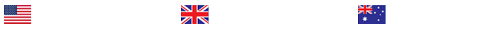Time Series (Statistics)

Time Series (Statistics)The cmort dataset contains the average weekly cardiovascular mortality in Los Angeles County for 1970-1979. The data for the last year (1979) is only for the first 40 weeks. Therefore, we will analyze the data for 1970-1978. We can get this data using the following R code:library(astsa)x = as.vector(cmort[1:468])t = as.vector(time(cmort)[1:468])plot(t, x, type=”l”)We will remove the seasonal component from the data using the moving average technique.First choose an appropriate value of the period d and find the pre-estimate of mt . Plot the observed series in gray and show the pre-estimate on the same plot but in black (be sure to plot your time series using the type = “l” command).Now find the estimate of st and plot these estimates for all t = 1, . . . , 468.Find the residuals after removing the seasonal component and plot them.Comment on whether you think these residuals look stationary or not. If they do not look stationary, what should we do to make them “look” stationary?require(datasets)X = as.vector(USAccDeaths)t = 1:length(X)plot(USAccDeaths, type = “o”, ylab = “Accidental Deaths”, col=”darkgray”)mav.even <- function(x,d=12){filter(x,c(0.5/d,rep(1/d,(d-1)),0.5/d), sides=2)}lines(as.vector(time(USAccDeaths)),mav.even(X), col = “black”)x = X – mav.even(X)w = unname(tapply(x, (seq_along(x)) %% 12, function(a) mean(a,na.rm = T)))w = w-mean(w)s = rep(w, 6)plot(as.vector(time(USAccDeaths)), s, type = “o”, ylab = “”, xlab = “”)d = X – splot(as.vector(time(USAccDeaths)), d, type = “o”, ylab = “”, xlab = “”)t = as.vector(time(USAccDeaths))w1 = tw2 = t^2fit = lm(d ~ w1+w2)lines(t, fitted(fit), type = “l”)plot(t,residuals(fit), type = “o”, ylab = bquote(hat(Y)[t]))

### Last Completed Projects

# topic title discipline academic level pages delivered
6
Writer's choice
University
2
1 hour 32 min
7
Wise Approach to
Philosophy
College
2
2 hours 19 min
8
1980's and 1990
History
College
3
2 hours 20 min
9
pick the best topic
Finance
School
2
2 hours 27 min
10
finance for leisure
Finance
University
12
2 hours 36 min